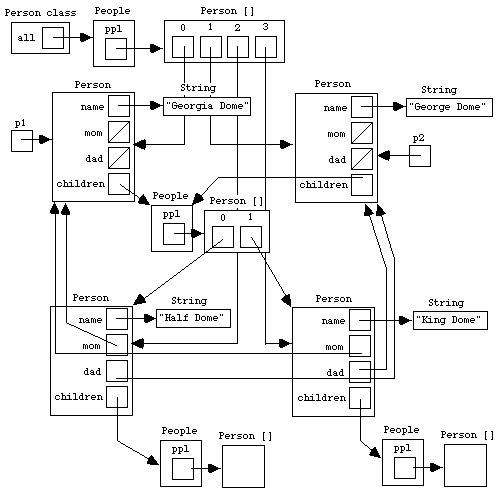Graphic by Keith Ohlfs CS111, Wellesley College, Fall 1997 Object Diagrams
[CS111 Home Page] [Syllabus] [Students] [Lecture Notes] [Assignments] [Programs] [Documentation] [Software Installation] [FAQ] [CS Dept.] [CWIS]

This document explains the rules for drawing object diagrams and then illustrates the rules in the context of a non-trivial example.

## Object Diagram Rules

Object diagrams consists of three kinds of entities: variables, objects, and classes. These are described below.

### Variables

A variable is a named location that is depicted as a name next to a box. The box may contain a primitive data type element (i.e., an element of type int, boolean, char, double, and a few other types) or a reference to an object. For instance: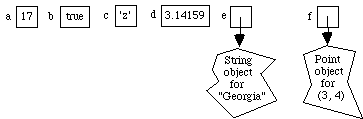Variables are created in Java via one of the following two declaration statements:

```type name;
type name = initial-value-expression;```

The first form creates a variable with a default value for the specified type. The second form creates a variable that holds the value of initial-value-expression, whose type must be type. For example, the above variables can be created by the following statements:

```int a = 17;
boolean b = true;
char c = 'z';
double d = 3.14159;
String e = "Georgia";
Point f = new Point(3,4);```

Important :

• A Java variable never has other boxes within it. It may contain a pointer to an object, but never contain the object itself.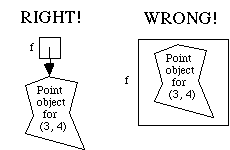• An object diagram never contains a pointer to a variable. For instance, the following picture is non-sensical in Java: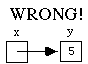There are four kinds of variables:

1. Local variables are those declared in method bodies; these appear in the variable portion of a Java execution frame. Unless explicitly requested, you generally need not show execution frames in an object diagram.
2. Instance variables are non-static variables declared within a class body; these appear within an instance object box; see the discussion of objects below.
3. Class variables are static variables declared within a class body; these appear within a class box; see the discussion of classes below;
4. Indexed variables are numbered variables that appear within array objects; see the discussion of array objects below.

### Objects

A Java object is a collection of variables. It is depicted as a labelled box containing its component variables. The label indicates which kinds of object it is. There are two kinds of objects: instance objects and array objects.

#### Instance Objects

An instance object is an object constructed from the template of a particular class. The variables within an instance object are the instance variables of the class. The label of an instance object is the name of the class from which the instance was derived.

Suppose the Square class has the following instance variables:

• An integer side specifying the side length of the square.
• A Point object corner specifying the upper left-hand corner of the square in a two dimensional grid.
• A boolean isFilled specifying whether the square should be drawn filled or not.

Here is the picture for an instance of a Square class: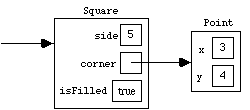Java programs never manipulate the instance box itself but only references (pointers) to the instance box. So technically we should say the "the corner variable contains a reference to a Point object". However, it is common to identify the reference to the object with the object itself, so we will often use the looser language "the corner variable contains a Point object". But it is important to keep in mind that a varable box can never contain subboxes in Java; it can only contain a reference to a box that contains variable boxes.

Because objects can never be manipulated without a reference to them, we will always draw objects as boxes with at least one reference to them, even though that reference may not be contained by any variable. The above diagram shows such a "free-floating" reference to the instance of the Square class.

In an object diagram, there may be many references to a particular instance box; all such references are considered to refer to the exact same object. For example, in the following picture, variables a and b contain the same Square object while c contains a different Square object. Both Square objects contain the same corner Point object.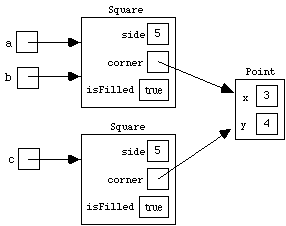The fact that object diagrams can show that the same object is "shared" by several variables is critical to understanding computation in Java. Sharing can be observed when an object is mutatated -- that is, when the values of its instance variables change. For example, if the side of the Square contained in a is changed to 6, then the Square in b is also changed but the Square in c is not. If the x coordinate of the Point in the above diagram is changed to 7, then the Squares in a, b, and c all can "see" this change.

Even without mutation, sharing can be detected in Java by the equality operator ==. When == is applied to two objects references, it is only true if they are refereces to the same object. For example, in the above diagram, (a == b) is true but (a == c) and (b == c) are false. If the corner of a square can be extracted by a getCorner() method, then all of the following are true:

```(a.getCorner() == b.getCorner())
(a.getCorner() == c.getCorner())
(b.getCorner() == c.getCorner())```

The instance variables of a class are specified within a class declaration. Here is a skeleton of the class declaration for the Square class:

```class Square {

// Instance variables;
private int side;
private Point corner;
private boolean isFilled;

// Constructor Method
public Square (i, p, b) {
side = i;
corner = p;
isFilled = b;
}

// Instance Methods go here.
...
}```

The private keywords in the instance variable declaration indicate that the variables can only be manipulated by the methods of the class; they cannot be directly extracted from the object.

Important: The only way to know what instance variables should be in an instance object is to examine the definition of the class of that instance.

Sometimes the class of an object is not available for inspection, in which case the private instance variables of the class cannot be determined. In this case, we must resort to a more abstract representation of the instance object. For instance, if we do not know that the Point class has instance variables named x and y, we can represent a Point instance with x-coordinate 3 and y-coordinate 4 as follows: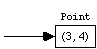This sort of abstract representation is commonly used for instances of the String class, whose implementation details are not available for inspection: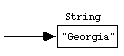Instances of a class are created by using new in conjuction with a constructor method for the class. Here is a sequence of statements that creates the objects shown in the two-square diagram above:

```Square a = new Square(5, new Point(3, 4), true);
Square b = a;
Square c = new Square(5, a.getCorner(), true);```

Note that the number of objects in the diagram (3) is exactly the number of new expressions that were executed. Since objects can only be created by new, this is always the case. (However, be careful: some uses of new are hidden. For instance, creating the String object "Georgia" involves a hidden use of new.)

Note that the corner of Square c is extracted from a via a.getCorner() (which is equivalent to b.getCorner()) -- this establishes the correct sharing shown by the diagram. It would be incorrect to write

`Square c = new Square(5, new Point(3,4), true);`

because this would create a second Point object, and there is only one in the diagram.

In many cases the object reference that belongs in an variable is not known when the variable is created. In this case, a special so-called null reference (written in Java as null) can be stored in the variable. In fact, the null reference is the default value for a variable that contains an object. In an object diagram, a variable containing the null reference is drawn as a box with a slash; for example: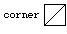#### Array Objects

An array object is an object containing a specified number of so-called indexed variables that are all of the same type. The number of variables in the array object is the length of the array. The indexed variables are named by integers between 0 and length - 1 inclusive.

An array object is depicted as a box containing numbered variables. The box has the label Type [ ], where Type is the type of the variables contained in the array. For example, here is an integer array , a point array, and an array of integer arrays: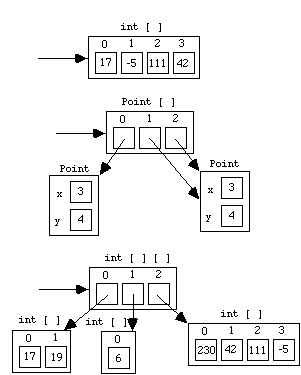As with instance objects, array objects are always manipulated by references (pointers) to the array object, and we commonly identify the pointer with the array object itself. As with instances, references are critical for exhibiting any sharing involved in a collection of array objects. For example, in the following diagram, Georgia's scores array is the same object as the array g, but Abby's scores array is a different array that happens to have the same values as Georgia's.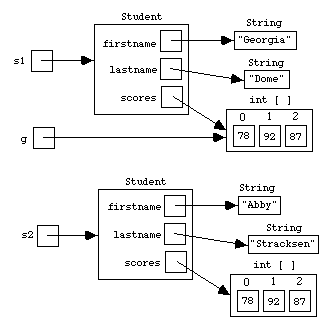If exp is any expression that denotes a reference to an array object and num is any expression that denotes an integer i, then exp[num] names the variable with label i in array denoted by exp. For instance, g in the above example names variable 0 of array g. As with other variables, this notation refers to the variable box when it appears on the left hand side of an assignment (as in g = 83) and refers to the contents of the variable box in all other contexts (as in g + 3). Note: the array expression need not be a variable name. For example, if the Student class supports a getScores() method, then the following is a meaningful statement:

s1.getScores() = s2.getScores() + 5;

The length of an array object is extracted via the .length syntax: E.g., g.length, s1.getScores().length.

An array object of length n with variables of type type is created via new type[n]. For example, the Student diagram above could be created by the following statements (assuming an appropriately defined Student class):

```g = new int;
g = 78;
g = 92;
g = 87;

s1 = new Student("Georgia", "Dome", g);

s2 = new Student("Abby", "Stracksen", newArray);
s2.getScores() = 78;
s2.getScores() = 92;
s2.getScores() = 87;```

### Classes

Like instance objects, Java classes themselves can have variables (called class variables) and methods (called class methods). In fact, in many object-oriented languages, classes are themselves a kind of object (usually instances of a class named Class!). However, in Java, classes are not objects. They are "second-class citizens" of the Java world. They have names, but there are no references (pointers) to classes. So it is not possible to store a class in a variable, or pass it as a parameter to a method.

In object diagrams, a class named class-name will be depicted as a box labelled class-name class. The box contains the class variables of the class. For example, suppose that the Student class keeps track of the number of students and the last student created. Then a box for the Student class could be added to the above diagram as shown below.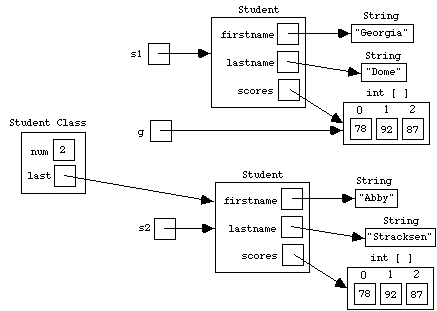Important: An object diagram should never show a reference (pointer) to a class.

Class variables are tagged by the keyword static within a class declaration. For example, the above diagram implies that the declaration of the Student class matches the following skeleton:

```class Student {

// Class variables
private static int num = 0;
private static Student last = null;

// Instance variables
private String firstname;
private String lastname;
private int [ ] scores;

// Constructor method
public Student (String fst, String lst, int [ ] scores) {
firstname = fst;
lastname = lst;
this.scores = scores;
num = num + 1;
last = this;
}

// Class methods
...

// Instances methods
...

}```

## Example

In this section we explore object diagrams in the context of a non-trivial example. The example is based on the Person and People classes shown below:

```public class Person {

// Class variable
private static People all = new People();

// Instance variables
private String name;
private Person mom;
private People children;

// Constructor Method
public Person (String name, Person mom, Person dad) {
this.name = name;
this.mom = mom;
this.children = new People();
}

public static People allPeople() {
return all;
}

public static void test() {
Person p1 = new Person("Georgia Dome", null, null);
Person p2 = new Person("George Dome", null, null);
p2.setChildren(p1.getChildren());
Person.allPeople().println();
}

public static void main (String [ ] args) {
test();
}

// Instance Methods

public String getName() {
return name;
}

public Person getMom() {
return mom;
}

}

public People getChildren() {
return children;
}

public void setChildren(People p) {
children = p;
}

}

public void println() {
System.out.println(name);
System.out.println("  Mom: " + ((mom==null) ? "unknown" : mom.getName()));
System.out.println("  Children: " + children.names());
}
}

class People {

// Instance variable
private Person [ ] ppl;

// Constructor method
public People() {
ppl = new Person;
}

// Instance methods
Person [ ] newPpl = new Person [ppl.length+1];
for (int i = 0; i < ppl.length; i++) {
newPpl[i] = ppl[i];
}
newPpl[ppl.length] = p;
ppl = newPpl;
}

public void println() {
for (int i = 0; i < ppl.length; i++) {
ppl[i].println();
}
}

public String names() {
// Return a string of the names in people
StringBuffer sb = new StringBuffer();
if (ppl.length > 0) {
sb.append(ppl.getName());
}
for (int i = 1; i < ppl.length; i++) {
sb.append(", ");
sb.append(ppl[i].getName());
}
return sb.toString();
}
}```

The class method invocation People.test() creates the object diagram shown below. You should work out the details of the object diagram on your own and compare your solution to the official one. There are a number of details worth mentioning:

• The "free-floating" variables p1 and p2 are local variables from the execution frame for People.test(); this execution frame is not shown in the diagram.
• The Georgia Dome and George Dome Person objects share the same People object for their children.
• The People objects for the children of Half Dome and King Dome both point to empty Person arrays. However, since these arrays are created by distinct calls to new, the two arrays (as well as the two empty People objects) are not equal via ==.
• The example highlights that instances of an object can point to other instances of an object. In fact, a path of such object references can lead back to itself to form a cycle. For example, the Georgia Dome Person object indirectly points to the Half Dome Person object through the children instance variable, while the Half Dome Person object directly points to the Georgia Dome Person object via the mom instance variable. Such cycles are essential for modelling mutual dependencies among objects and are common in real programs.